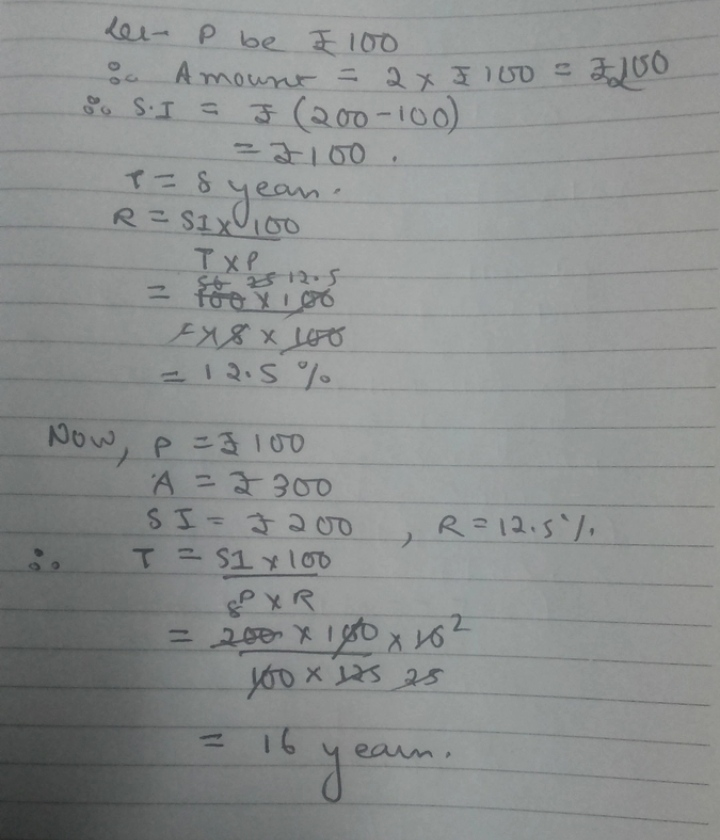×#### Thank you for registering.

One of our academic counsellors will contact you within 1 working day.

Click to Chat

1800-1023-196

+91-120-4616500

CART 0

• 0

MY CART (5)

Use Coupon: CART20 and get 20% off on all online Study Material

ITEM
DETAILS
MRP
DISCOUNT
FINAL PRICE
Total Price: Rs.

There are no items in this cart.
Continue Shopping
```
A certain sum of money doubles itself at simple interest in 8 years. In how many years will it be three times at the same rate

```
8 months agoSaurabh Koranglekar
10233 Points
``````
8 months ago
```							Sum of money = pInterest = r%Time1 = 8yTime2 = t Hence, p×r×8/100 = p [As the money doubles, interest accumulated will be same as the amount of money].=> r=12.5 In the second case, p×r×t/100 = 2p [3p = p+2p(interest)]=> t = 200/r = 16. Hence, required time = 16y.
```
7 months ago
```							Let the principle be 100.Then amount will be 200.Interest= 100Time = 8 yrsR=?I=PRT/100100=100*R*8/100100*100/100*8=R100/8=RNOW SUM SHOULD BE 4 TIMES MEANS 400MEANS INTREST ON 100 IS 300.I=PRT/100300=100*100/8*T/100300*8/100=T24= TIN 24 YEARS SUM WILL BECOME 4 TIMES OF PRINCIPLE
```
6 months ago
Think You Can Provide A Better Answer ?

## Other Related Questions on 7 grade maths

View all Questions »### Course Features

• 728 Video Lectures
• Revision Notes
• Previous Year Papers
• Mind Map
• Study Planner
• NCERT Solutions
• Discussion Forum
• Test paper with Video Solution### Course Features

• 731 Video Lectures
• Revision Notes
• Test paper with Video Solution
• Mind Map
• Study Planner
• NCERT Solutions
• Discussion Forum
• Previous Year Exam Questions